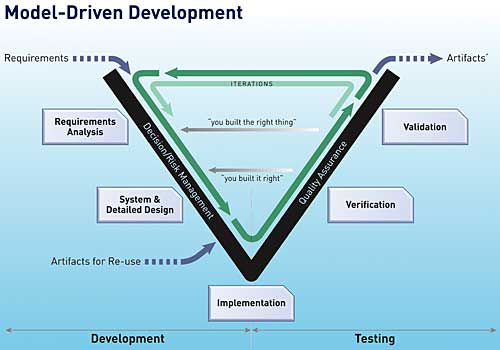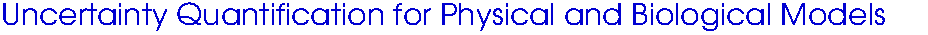Course Information Help Local linksBackground and Motivation Lecture 1: Motivation and Prototypical Examples (PDF) Probability and Statistics Background Lectures 2-4: Statistical models and interval estimators (PDF) Supplemental Material: Random variables, estimators and sampling distributions (PDF) Parameter Selection Techniques Lectures 5-8: Local and Global Sensitivity Analysis (PDF) Complex Step Derivative Approximations (PDF) Lecture 9: Active subspaces (PDF) Statistical Model Calibration Lectures 10-11: Frequentist techniques for model calibration (PDF) Lectures 12-14: Bayesian techniques for model calibration: Part 1 (PDF) Lectures 15-16: Bayesian techniques for model calibration: Part 2 (PDF) Uncertainty Propagation in Models Lectures 17-18: Uncertainty Propagation (PDF) Surrogate Models Lectures 19-21: Numerical Surrugate Models (PDF) Lectures 12-23: Statistical Surrugate Models (PDF) Lectures 24-25 Numerical Surrugate Models for PDE (PDF) Model Discrepancy and Active Subspace-Based Inference Lecture 29: Model discrepancey (PDF) Lecture 29: Active subspace-based Bayesian inference (PDF)